# Introduction and Properties of Minimum Spanning Tree

## Introduction

We have probably already learned about graphs. There we saw what a tree is, but have you ever heard of a spanning tree?

Probably not, so let’s see what it is!

We already know that a graph consists of a set of vertices and edges like this:

Here the set of vertices is V = {1, 2, 3, 4, 5, 6, 7, 8}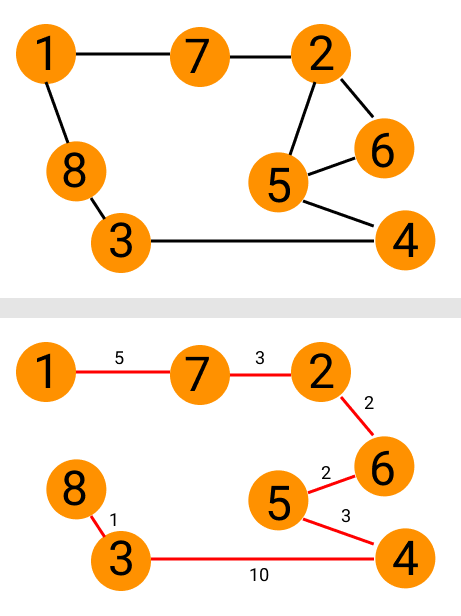and, the set of edges is E = {(1,2), (2,3), (3,4), (4,5), (5,6), (6,7), (7,8), (8,1)}

## Spanning Trees

To obtain a spanning tree from the above graph, we’ll have to make a tree taking all the vertices of the graph and choosing only (N-1) edges from the tree. Here, N is the total number of vertices in the graph.

The only thing we need to make sure of is, all the vertices must be connected and it satisfies all the properties of a tree. A graph can have multiple spanning trees.

For example, a spanning tree from the above graph will be: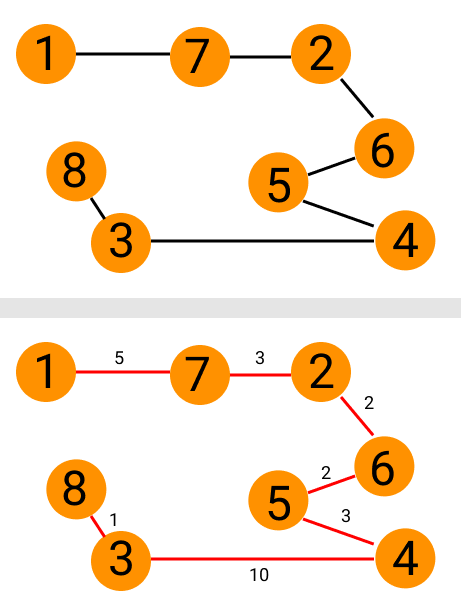Thus, to formally define it,

A spanning tree is a connected and undirected graph (n - 1) number of edges, where n is the number of vertices.

## Minimum Spanning Tree

Now, let’s consider a weighted graph.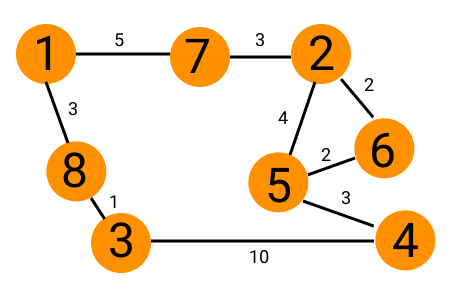We can find different spanning trees, each of which will have different weights for different paths. This sum of the weights is known as cost.

For example, the weight of the spanning tree shown below is 26.

Similarly, there are many other combinations of spanning trees possible.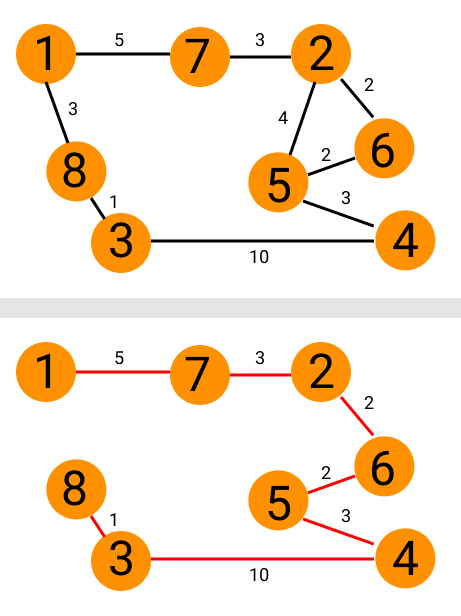Now, suppose that the paths in a spanning tree are the different routes from one place to another. Which road will we prefer?Source: giphy

The shortest one, of course! So, we need to find the path with the least weight. This is called a minimum spanning tree

Let’s move on to the properties of a minimum spanning tree.

## Properties of Minimum Spanning Tree

Some of the important properties of minimum spanning tree are as follows:

### Possible Multiplicity

We already saw that by the definition of a spanning tree, it must have (n - 1) edges where n is the number of vertices in the graph. This property of a minimum spanning tree is formally called possible multiplicity.

### Uniqueness

According to this property, if all the edges in a spanning tree have different weights, there will be only one unique minimum spanning tree.

Let us see an example of this property.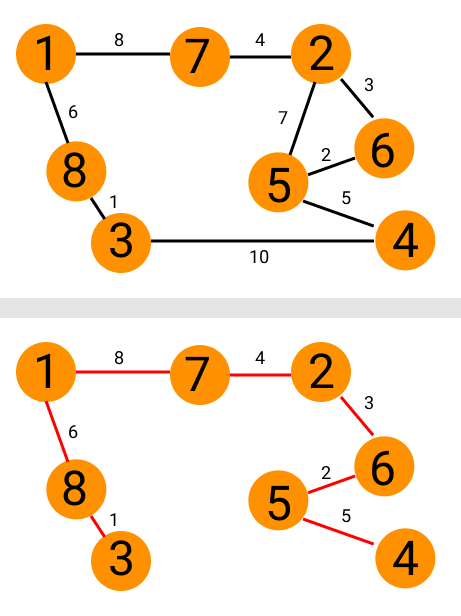The graph  The minimum spanning tree

### Minimum Cost Subgraph

This property states that if the weights of all the edges in the spanning tree are positive, then the minimum spanning tree will also be a minimum cost subgraph that connects all the vertices.

To understand this point, let us consider our previous example again.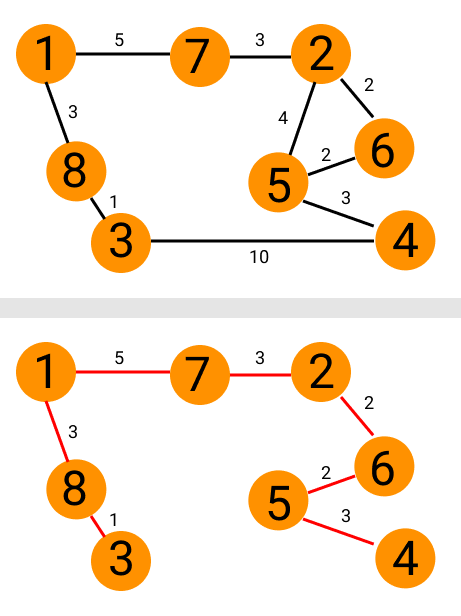The graph and its minimum spanning tree.

If you notice, it is also the minimum cost sub-graph for the given graph.

### Minimum Cost Edge

By this property, if the edge with the least weight is present only once, it must be included in the minimum spanning tree. We saw that in our example practically too, where the edge with the least weight (= 1) is unique and is present in the minimum spanning tree.Graph   MinimumSpanning Tree

### Cycle Property

In the graph below, we can see a cycle between nodes 2, 6 and 5. In this cycle, the weights of the edges are 2, 2 and 4, respectively.

The edge between nodes 2 and 5 has the largest weight (= 4) out of these edges.

So, according to the cycle property, this edge will not be a part of the minimum spanning tree.Thus, the cycle property states that in a cycle, the edge with the largest weight will never be a part of the minimum spanning tree

### Cut Property

To understand the cut property, let us first cut our graph as shown below.

Here, the edges we are cutting through (also known as cut edges) are having weights 3, 4 and 2. Out of these edges, the edge with the least weight is 2.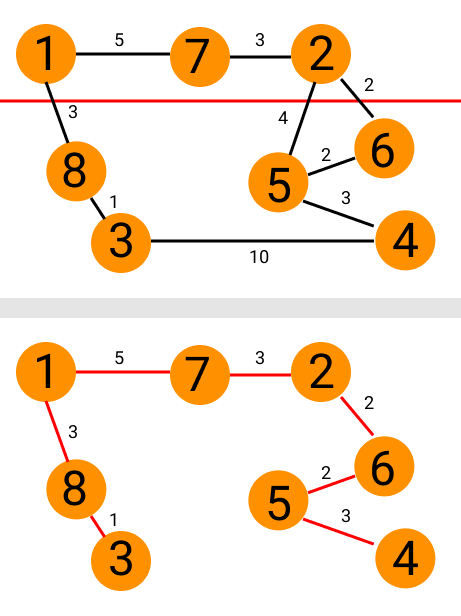So, by the cut property, this edge will be a part of the minimum spanning tree.

Thus, by the cut property, if we cut a graph, the cutting edge with the least weight will always be a part of the minimum spanning tree.

These were all the crucial properties of a minimum spanning tree, let’s move on to some of the frequently asked questions of this topic.

Q1) What is a minimum spanning tree?

A1) A spanning tree is a connected and undirected graph (n - 1) number of edges, where n is the number of vertices. If the edges have weights, the path with the least sum of weights is called the minimum spanning tree.

Q2)What are the properties of a minimum spanning tree?

A2)The properties of an MST are:

• Possible Multiplicity
• Uniqueness
• Minimum Cost Subgraph
• Minimum Cost Edge
• Cycle Property
• Cut Property
• Contraction

Q3)What does the possible multiplicity of an MST mean?

A3)Possible multiplicity means that an MST will have (n - 1) edges where n is the number of vertices in the graph.

Q4)State the cycle property and the cut property of an MST.

A4)The cycle property states that in a cycle, the edge with the largest weight will never be a part of an MST.

By the cut property, if we cut a graph, the cutting edge with the least weight will always be a part of the minimum spanning tree.

Q5)What are some applications of an MST?

A5)Some applications of an MST are:

• Taxonomy
• Designing networks
• Circuit design
• To describe financial markets

## Key Takeaways

In this article, we learned what a minimum spanning tree is and what its different properties are. Now that we are well versed with the theoretical knowledge of minimum spanning trees, we should know how to find them from a given graph. To do that, there are two algorithms, Kruskal’s algorithm and Prim’s algorithm

Apart from this, there are many other algorithms that we need to learn and practice. CodeStudio is a platform where you can find practice coding questions and the interview experience of people working in reputed companies to further your knowledge.

Happy Learning!